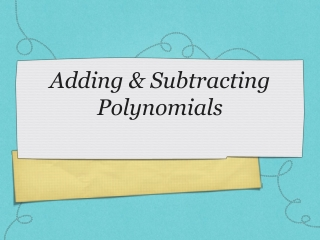DownloadDownload PresentationTélécharger la présentation- - - - - - - - - - - - - - - - - - - - - - - - - - - E N D - - - - - - - - - - - - - - - - - - - - - - - - - - -
##### Presentation Transcript

2. What is a Polynomial? • View this Cool Math lesson introducing Polynomials. • There is only the 1 page of notes.

3. Describe Polynomials • View this Cool Math lesson introducing terms describing Polynomials. • There are 3 pages of notes. • Be sure to complete the Your Turnproblems in your notebook. Check your answers on the next slides.

4. Your Turn on Page 3 • Find the degree of 3x - x2. • The degree is 2. • Find the degree ofa4b - 7a2b2 . • The degree is 5. • Find the degree of7x - 1. • The degree is 1.

5. Adding & Subtracting Polynomials • View this Cool Math lesson to learn how to add and subtract polynomials. • There are 5 pages of notes. • Be sure to complete the Try It and Your Turnproblems in your notebook. Check your answers on the next slides.

6. Try It on Page 2 • Simplify: Remove parenthesis. Rearrange so like terms are together. Combine like terms.

7. Your Turn on Page 3 • Simplify. Remove parenthesis. Rearrange so like terms are together. Combine like terms.

8. Try It on Page 3 • Simplify. Remove parenthesis. Rearrange so like terms are together. Combine like terms.

9. Your Turn on Page 4 • Simplify. Remove parenthesis but distribute the negative. Rearrange so like terms are together. Combine like terms.

10. Your Turn on Page 5 Write as a subtraction. Remove parenthesis but distribute the negative. Rearrange so like terms are together. Combine like terms.

11. Gizmo Practice Activity • Let's practice some more at the Explore Learning website using the username and password given to you AND found in the support documents. • Search for the "Addition of Polynomials - Activity A" Gizmo, launch the Gizmo and try at least 5 problems. • After you have completed at least 5 Gizmo problems, scroll down to the Assessment Questions and complete the 4 multiple choice problems. • Pronto or Message me if you have questions.